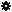﻿ Object Model: NurbsSurface.GetNormalizedUVFromUV2

NurbsSurface.GetNormalizedUVFromUV2

## NurbsSurface.GetNormalizedUVFromUV2### Description

Returns the Normalized equivalent of UV values. The data is returned in a 1-dimensional array and is ordered the same as for the NurbsSurface.GetNormalizedUVFromUV method output arguments.

Note: This method must be used with scripting languages that don't support arguments passed by reference such as JScript. For more information on getting output arguments, see About Output Argument Arrays.

### C# Syntax

 ```Object NurbsSurface.GetNormalizedUVFromUV2( Double in_dUValue, Double in_dVValue ); ```

### Scripting Syntax

 ```oReturn = NurbsSurface.GetNormalizedUVFromUV2( UValue, VValue ); ```

### Return Value

Array of normalized values ordered as UValueNormalized, VValueNormalized.

### Parameters

Parameter Type Description
UValue Double The UValue from which we want the normalized UValue.
VValue Double The VValue from which we want the normalized VValue.

### Examples

#### JScript Example

 ```/* This example shows how to retrieve the normalized equivalent of the UV values: 3.0, 4.0; from NurbsSurface.GetNormalizedUVFromUV2 */ var oRoot = Application.ActiveProject.ActiveScene.Root; var oSphere = oRoot.AddGeometry( "Sphere", "NurbsSurface" ); // Convert VB array to JScript array var vbArgs = new VBArray(oSphere.ActivePrimitive.Geometry.Surfaces(0).GetNormalizedUVFromUV2( 3.0, 4.0 )); var args = vbArgs.toArray(); // Get the U normalized value Application.LogMessage("U normalized value: " + args ); // Get the V normalized value Application.LogMessage("V normalized value: " + args ); ```